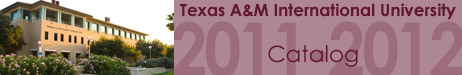Home >> Course Descriptions >> College of Arts and Sciences MATH 5340 Differential Geometry Three semester hours. This course is an introduction to the differential geometry of n-dimensional manifolds. Topics include: Riemannian manifolds, differential forms and Stokes Theorem. (Note that differential geometry is relevant to differential equations, mathematical physics, as well as other areas of sciences.) Prerequisites: MATH 5365 and knowledge equivalent to MATH 2415 and MATH 4335.